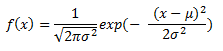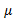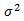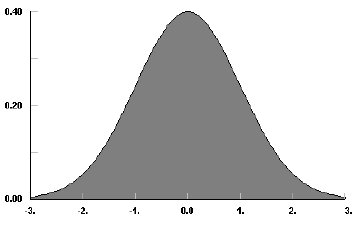AnyLogic

# normal

 DensityMeanVarianceModeThe Normal distribution is an unbounded continuous distribution. It is sometimes called a Gaussian distribution or the bell curve. Because of its property of representing an increasing sum of small, independent errors, the Normal distribution finds many, many uses in statistics. It is wrongly used in many situations. Possibly, the most important test in the fitting of analytical distributions is the elimination of the Normal distribution as a possible candidate.

The Normal distribution is used as an approximation for the Binomial distribution when the values of n, p are in the appropriate range. The Normal distribution is frequently used to represent symmetrical data, but suffers from being unbounded in both directions. If the data is known to have a lower bound, it may be better represented by suitable parameterization of the Lognormal, Weibull or Gamma distributions. If the data is known to have both upper and lower bounds, the Beta distribution can be used, although much work has been done on truncated Normal distributions.

#### Example

sigma =1; mean = 0### normal(double sigma, double mean)

Description
Generates a sample of the Normal distribution.
Parameters
Name Type of value Description
sigma double The shape parameter = standard deviation.
mean double The shift parameter = mean value.
Result
Type Description
double The generated sample.

### normal(double sigma)

Description
Generates a sample of the Normal distribution with mean set to 0. Is equivalent to normal(sigma, 0).
Parameters
Name Type of value Description
sigma double The shape parameter = standard deviation.
Result
Type Description
double The generated sample.

### normal()

Description
Generates a sample of the Normal distribution with mean set to 0 and sigma set to 1. Is equivalent to normal(1, 0).
Result
Type Description
double The generated sample.

### normal(double sigma, mean,java.util.Random r)

Description
Generates a sample of the Normal distribution using the specified random number generator.
Parameters
Name Type of value Description
sigma double The shape parameter = standard deviation.
mean double The shift parameter = mean value.
r java.util.Random The random number generator.
Result
Type Description
double The generated sample.

This document includes content from the “Stat::Fit User's Manual”. Copyright 2016 Geer Mountain Software Corp.